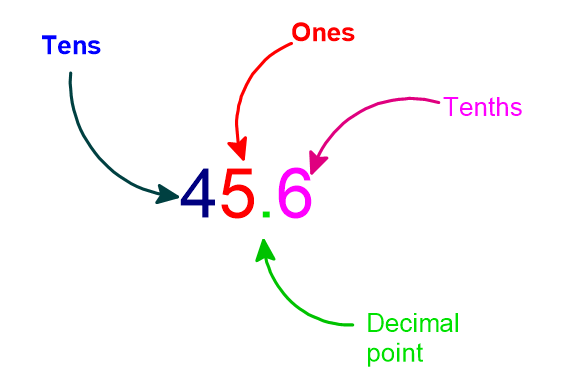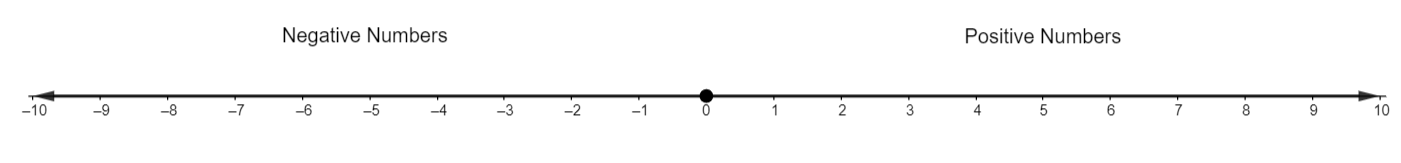Get instant Arithmetic help from the best tutors at TutorEye

# Math assignment to be done? Get instant arithmetic help at TutorEye!

Have a Math assignment waiting to be solved? We have the best tutors at TutorEye to help you. Get in touch now!

## Top Questions

t schedule 3 people for the day my % scheduled is 78.57% My question is how did the computer come up with the 78.57%?
View More

I would love to get a written solution. I did the other proof tree that you can see in the attaached files. So its supposed to look like that Thank You.
View More

xtbook. i really need in in half an hour
View More

## Arithmetic Basic Operations

### Natural Numbers:

The set of non-negative counting numbers. Example: {1, 2, 3, 4, 5 ...}.

Sometimes, a set of non-negative whole numbers is also called natural numbers. Example: {0, 1, 2, 3, 4,  ...}

It does not contain any fractions.

### Even & Odd Numbers:

If an integer is perfectly divided by 2. Then it is an even number. The last digit would be 0, 2, 4, 6, or 8. Example: -12, 0, 6, and 38.

And, if an integer is not perfectly divided by 2. Then that is an odd number. The last digit would be 1, 3, 5, 7, or 9. Example: -15, 1, 9, and 33.

### Laws of Exponents:

The exponent of a number means how many times we repeat the multiplication of the same number by itself. Example:An exponent with a negative sign means division. Example:And, an exponent with fractionwould be theroot. Example:Some other important rules are:### Multiplication and Division:

The basic idea of multiplication is adding the numbers by repeating them. Example:.

The division is the opposite of multiplication. Here, we split the numbers into equal parts. Example:.

### Decimals:

The position of the decimal point will always be between Ones and Tenths.Moving the decimal point to the left by one digit means a multiplication of 10. Example:Shifting the decimal point to the right by one digit means a division by 10. Example:.

### Comparing Fractions:

Comparing the two fractions can be done by converting the fraction into a decimal or making the denominator identical, if not already the same.

### Percentages:

The percent means the value corresponds to “per 100”. It is represented by the symbol %. And, the percentage is the product of percent and a quantity.

In other words, a percentage is a part per 100. However, in practice, both percent and percentage are used in the same way.

### Ratios and Proportion:

The ratio is used to compare two quantities. The ratio of a to b is represented byor.

Proportion is a term representing that two ratios are equal. The proportion of quantities a,b,c,d can be represented by using a fraction asor, using a colon.

### Like and Unlike Fractions:

Like fraction is the group of fractions that have an identical denominator. Example:.

And, the group of fractions that do not have the identical denominator is called, unlike fractions. Example:.

### Surds:

Surds are the value in the root, which can not be accurately written as a fraction and when simplified, it will have a non-repeating decimal. They are also called irrational numbers. Example:.

### Sum of n Terms:

The sum of n terms of a natural number under arithmetic progression can be written as:In an arithmetic progression, whose first term is a and the common difference is d, the sum can be calculated using one of the following formulas:### Integers:

The set of integers are positive and negative whole numbers including zero. And, it does not have any fraction.

### Geometric Sequence:

In a geometric sequence, every term is multiplied by a constant ratio called the common ratio.

Example: {1, 3, 9, 27, …}. In this case, the common ratio is 3.

### Fundamental Theorem:

Any positive integer which is greater than one is either a prime number or is the product of one or more prime number in exactly one way, ignoring the rearrangement.

Example:.

### Fraction to Decimal

A fraction can be converted into a decimal by simply dividing the numerator by the denominator using long division.

Example:### Variable:

In an equation, a variable is a symbol that is used to represent an unknown value.

Example:, where x and y are variable.

### Number Line:

It is a pictorial representation of numbers on a line, numbers are placed after an equal interval.### Whole Number:

The set of numbers that have all positive numbers including zero. Whole numbers do not include decimals, fractions.

Example: {0, 1, 2, 3, 4, 5 ...}.

### Even and Odd Numbers:

An integer that can be perfectly divided by 2 is called an even number. The last digit will always consist of either 0, 2, 4, 6 or, 8.

And, an odd number is an integer that can not be perfectly divided by 2. The last digit would always consist of 1, 3, 5, 7 or, 9.

### Word problems on Decimals:

Example 1: The average height of the students participating in a basketball match is, and the height of their trainer is. Calculate the difference of their height in cm?

Example 2: The cost of purchasing a pencil isif you purchase 6 pencils and you have. How much money will you have after purchasing those pencils?

### Integers on a Number Line

Integers present on the left side of zero are negative, while the right side integers are positive. Also, each number is placed after a fixed interval.Some of the common arithmetic advanced operations are computation of percentage, exponentiation, square root, etc.

### Computation of percentages:

The percent means the value corresponds to “per 100”. If we want to calculate the P % of a value X, we could calculate using the following formula.

Let us say, Y is the P % of a value X. Hence,### Logarithmic Functions:

The general logarithmic function is, where base, and. If the base is 10, we write it as, and when the base is Euler’s number e. It would be called the natural logarithm,.

### Exponentiation:

The general form of an exponential function is, where a is the base and is a constant value, and x is the variable.

When the base is Euler’s number e. It would. This is the commonly used exponential function.

### Square roots:

The square root of a value is a number, which when multiplied by itself, gives the value.

There is a mathematical symbol to represent square root,This symbol is called radical. The square root of a perfect square is easy to calculate.

## Sample High School Arithmetic Problems

Question 1: Calculate the 5% of 60:

Explanation: Let us say, Y is the 5 % of value 60.

Using the percent formula,Now, plug in the value, we get.Answer: 5% of 60 would be 3.

Question 2: Calculate the square root of 64.

Explanation: Let us say, x is the square root of 64. We could rewrite it as.

Now, we need to factor 64 in pairs, such that both numbers are the same.

So,. Now, we have a number 8, which when multiplied by itself, gives 64.

Answer: Square root of 64 would be 8.

Question 3:CompareExplanation: To compare the fraction, we can either convert them into decimals or by making the denominator identical.

Let us write the decimal and compare.Answer: We can see,is greater than.

## Are you not good with the numbers? Here’s how you can improve your arithmetic skills

Arithmetic is something that finds the most practical uses even outside of your academic life. Look around and you’d find its prevalence in almost everything, varying from basic to advanced use.

Be it something as simple as buying groceries to paying the taxes, there is arithmetic involved in every process. Do you think you can afford to be ‘not-so-good’ at it?

Absolutely not! Sometimes it is difficult to deal with the numbers. Agreed! But there are ways to enhance your skills in the subject before you start dreading it and let it affect your scores.

For you to be good at arithmetic, you should start with the basics and lay a stronger foundation for more advanced concepts.

Start with the easy calculations and once you feel confident, move on to the next level. Once you are good at the basics, it would greatly improve your understanding of the more complex techniques.

One of the factors that can play a major role here is “practice”. The more you practice arithmetic problems, the better you keep getting at solving them. Consistency is the key here. So practice daily with us and the hard work will definitely reflect in your scores.

If the arithmetic problems still seem to be a hard task, getting tutoring aid always helps.

You can schedule personalized sessions with a tutor according to your time preference. It will help you clear your concepts better and also build more confidence. You get to ask as many questions as you want.

TutorEye gets you in touch with proficient tutors with whom you can have 1:1 sessions. They will assess your learning style and academic goals and provide you with specialized courses at hand.

## Here are steps to get Arithmetic help at TutorEye

TutorEye ensures well-written and plagiarism-free solutions to your assignments. We have expert tutors to provide you the best answers at a reasonable price.

Just follow these simple steps to hire a homework helper:

Step 2: Hire a Math expert to guide you through a difficult assignment after mutually agreeing to a price and submission deadline.

Step 3: Payment to be released from escrow, once you are completely satisfied with the solutions submitted.

Our assignment solvers are known to deliver the best quality work. The solutions will be easily understandable by you and will help you clear the concepts. Our solutions will never get you landed in any copyright issues because of their originality.

So, skip any second thoughts and reach out to us. Get started with your homework now!

Question 1: What are basic arithmetic skills?

Answer: Basic arithmetic skills help in the calculation of the amount, measurements, which includes addition, subtraction, multiplication, and division.

Question 2: How can we improve arithmetic?

Answer: To improve arithmetic, we need to be accurate in the basic operations of mathematics. And, we should know, how to distribute the value inside the parenthesis.

Secondly, it is really important to have an understanding of the formula used. We need to understand what does the variable used in the formula means, and how we can find those variables.

Question 3: What is the easiest way to learn arithmetic?

Answer: If we practice with focus under the right guidance, we can master any topic.

For arithmetic, having an understanding of multiplication and division of integers would help in learning.

For example, multiplication and division of the same sign will always be positive. And, multiplication and division of opposite signs would always give the negative value.

Question 4: How to solve arithmetic progression problems?

Answer: To solve the AP problem, first, we need to analyze the problem and figure out what value we need to find out? Do we need the sum of terms, do we need common differences, or does it ask to find any specific term?

Then, we need to think about the appropriate formula. And accordingly, we need to calculate the variables. Like, if it was asking to find the sum of n terms, then we have to calculate the first term and common difference.

After this, we need to plug the variable into the formula. And, solve that expression carefully. Following these procedures will minimize the chance of error.